## RD Sharma Class 8 Solutions Chapter 14 Compound Interest Ex 14.1

These Solutions are part of RD Sharma Class 8 Solutions. Here we have given RD Sharma Class 8 Solutions Chapter 14 Compound Interest Ex 14.1

Other Exercises

Question 1.
Find the compound interest when principal = Rs 3,000, rate = 5% per annum and time = 2 years.
Solution:
Principal (P) = Rs 3,000
Rate (R) = 5% p.a.
Period (T) = 2 years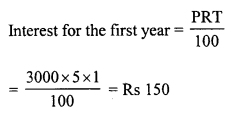Amount after one year = Rs 3,000 + Rs 150 = 3,150
and principal for the second year = Rs 3,150
and interest for the second year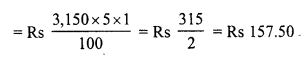Compound interest for two years = Rs 150 + Rs 157.50 = Rs 307.50

Question 2.
What will be the compound interest on Rs 4,000 in two years when rate of interest is 5% per annum ?
Solution:
Principal (P) = Rs 4,000
Rate (R) = 5% p.a.
Period (T) = 2 yearsAmount after one year = Rs 4,000 + Rs 200 = Rs 4,200
Principal for the second year = Rs 4,200
Interest for the second year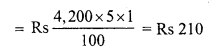Compound interest for 2 years = Rs 200 + Rs 210 = Rs 410

Question 3.
Rohit deposited Rs 8,000 with a finance company for 3 years at an interest of 15% per annum. What is the compound interest that Rohit gets after 3 years ?
Solution:
Principal (P) = Rs 8,000
Rate (R) = 15% p.a.
Period (T) = 3 years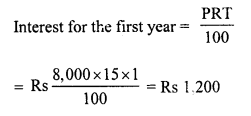Amount after first year = Rs 8,000 + RS 1,200 = Rs 9,200
or Principal for the second year = Rs 9,200
Interest for the second year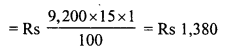Amount after 2 years = Rs 9,200 + Rs 1,380 = Rs 10,580
or Principal for the third year = Rs 10,580
Interest for the third yearCompound for the 3 years = Rs 1,200 + Rs 1,380 + Rs 1,587 = Rs 4,167

Question 4.
Find the compound interest on Rs 1,000 at the rate of 8% per annum for 1$$\frac { 1 }{ 2 }$$ years when interest is compounded half-yearly ?
Solution:
Principal (P) = Rs 1,000
Rate (R) = 8% p.a.
Period (T) = 1$$\frac { 1 }{ 2 }$$ years = 3 half-years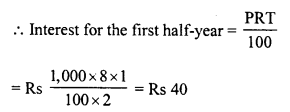Amount after one half-year = Rs 1,000 + Rs 40 = 1,040
Or principal for the second half-year = Rs 1,040
Interest for the second half-yearAmount after second half-year = Rs 1,040 + 41.60 = Rs 1,081.60
Or principal for the third half-year = Rs 1081.60
Interest for the third half-year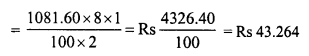Compound interest for the third half-years or 1$$\frac { 1 }{ 2 }$$ years
= Rs 40 + Rs 41.60 + Rs 43.264 = Rs 124.864

Question 5.
Find the compound interest on Rs 1,60,000 for one year at the rate of 20% per annum, if the interest is compounded quarterly.
Solution:
Principal (P) = Rs 1,60,000
Rate (R) = 20% p.a. or 5% quarterly
Period (T) = 1 year or 4 quartersAmount after first quarter = Rs 1,60,000 + 8,000 = 1,68,000
Or principal for the second quarter = Rs 1,68,000
Interest for the second quarterAmount after the second quarter = Rs 1,68,000 + Rs 8,400 = 1,76,400
Or principal for the third quarter = Rs 1,76,400
Interest for the third quarterAmount after third quarter = Rs 1,76,400 + 8,820 = Rs 1,85,220
or Principal for the fourth quarter = Rs. 1,85,220
Interest for the fourth quarter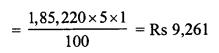Total compound interest for the 4 quarters = Rs 8,000 + Rs 8,400 + Rs 8,820 + 9,261 = Rs 34,481

Question 6.
Swati took a loan of Rs 16,000 against her insurance policy at the rate of 12$$\frac { 1 }{ 2 }$$ % per annum. Calculate the total compound interest payable by Swati after 3 years.
Solution:
Amount of loan or principal (P) = Rs 16,000Amount after first year = Rs 16,000 + Rs 2,000 = Rs 18,000
Principal for the second year = Rs 18,000
Interest for the second year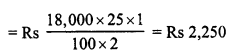Amount after second year = Rs 18,000 + 2,250 = Rs 20,250
Principal for the third year = Rs 20,250Compound for 3 years = Rs 2,000 + Rs 2,250 + 2531.25 = Rs 6,781.25

Question 7.
Roma borrowed Rs 64,000 from a bank for 1$$\frac { 1 }{ 2 }$$ years at the rate of 10% per annum. Compute the total compound interest payable by Roma after 1$$\frac { 1 }{ 2 }$$ years, if the interest is compounded half-yearly.
Solution:
Principal (sum borrowed) (P) = Rs 64,000
Rate (R) = 10% p.a. or 5% half-yearly
Period (T) = 1$$\frac { 1 }{ 2 }$$ years or 3 half-yearsAmount after first half-year = Rs 64,000 + Rs 3,200 = Rs 67,200
Or principal for the second half-year = Rs 67,200
Interest for the second half-yearAmount after second half-year = Rs 6,7200 + 3,360 = Rs 70,560
Or principal for the third half-year = Rs 70,560
Interest for the third half-year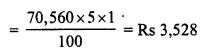Total compound interest for 3 half-years
or 1$$\frac { 1 }{ 2 }$$ years = Rs 3,200 + Rs 3,360 + Rs 3,528 = Rs 10,088

Question 8.
Mewa Lai borrowed Rs 20,000 from his friend RoopLal at 18% per annum simple interest. He lent it to Rampal at the same rate but compounded annually. Find his gain after 2 years.
Solution:
Principal (P) = Rs 20,000
Rate (R) = 18% p.a.
Period (T) = 2 years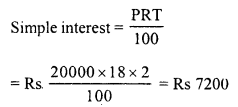In second case
Interest for the first yearAmount after one year = Rs 20,000 + Rs 3,600 = Rs 23,600
Or principal for the second year = Rs 23,600
Interest for the second yearInterest for two years = Rs 3,600 + 4,248 = Rs 7,848
Gain = Rs 7,848 – Rs 7,200 = Rs 648

Question 9.
Find the compound interest on Rs 8,000 for 9 months at 20% per annum compounded quarterly.
Solution:
Principal (P) = Rs 8,000
Rate (R) = 20% p.a. or 5% p.a. quarterly
Period (T) = 9 months or 3 quarters
Interest for the first quarterly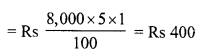Amount after first quarter = Rs 8,000 + Rs 400 = Rs 8,400
Or principal for second quarter = Rs 8,400
Interest for the second quarter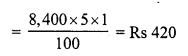Amount after second quarter = Rs 8,400 + Rs 420 = Rs 8,820
Or principal for the third quarter = Rs 8,820
Interest for the third quarterCompound interest for 9 months or 3 quarters = Rs 400 + Rs 420 + Rs 441 = Rs 1,261

Question 10.
Find the compound interest at the rate of 10% per annum for two years on that principal which in two years at the rate of 10% per annum gives Rs. 200 as simple interest.
Solution:
Simple interest = Rs 200
Rate (R) = 10% p.a.
Period (T) = 2 years.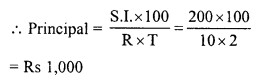Now in second case,
Principal CP) = Rs 1,000
Rate (R) = 10% p.a.
Period (T) = 2 years.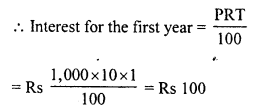Amount after one year = Rs 1,000+ Rs 100 = Rs 1,100
Or principal for the second year = Rs 1,100Total interest for two years = Rs 100 + Rs 110 = Rs 210

Question 11.
Find the compound interest on Rs 64,000 for 1 year at the rate of 10% per annum compounded quarterly.
Solution:
Principal (P) = Rs 64,000
Rate (R) = 10% p.a. or $$\frac { 5 }{ 2 }$$ % quarterly
Period (T) = 1 year = 4 quarters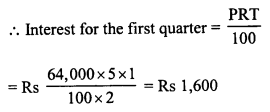Amount after first quarter = Rs 64,000 + Rs 1,600 = Rs 65,600
Or principal for the second quarter = Rs 65,600
Interest for the second quarter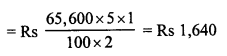Amount after second quarter = Rs 65,600 + Rs 1,640 = Rs 67,240
Or principal for the third year = Rs 67,240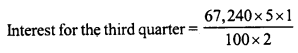= Rs 1,681
Amount after third quarter = Rs 67,240 + Rs 1,681 = Rs 68,921
Or principal for the fourth quarter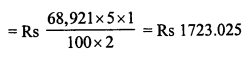Total compound interest for 4 quarters or one year
= Rs 1,600 + Rs 1,640 + Rs 1,681 + Rs 1723.025 = Rs 6644.025

Question 12.
Ramesh deposited Rs 7,500 in a bank which pays him 12% interest per annum compounded quarterly. What is the amount which he receives after 9 months ?
Solution:
Principal (P) = Rs 7,500
Rate (R) = 12% p.a. or 3% quarterly
Time (T) = 9 months or 3 quarters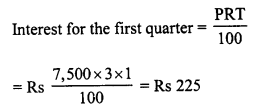Amount after one quarter = Rs 7,500 + Rs 225 = Rs 7,725
Or Principal for second quarter = Rs 7,725
Interest for the second quarter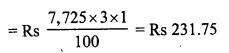Amount after second quarter = Rs 7,725 + Rs 231.75 = Rs 7956.75
Or principal for the third quarterTotal amount he received after 9 months = Rs 7956.75 + Rs 238.70 = Rs 8195.45

Question 13.
Anil borrowed a sum of Rs 9,600 to install a hand pump in his dairy. If the rate of interest is 5$$\frac { 1 }{ 2 }$$ % .per annum compounded annually, determine the compound interest which Anil will have to pay after 3 years.
Solution:
Principal (P) = Rs 9,600
Rate of interest (R) = 5$$\frac { 1 }{ 2 }$$ % = $$\frac { 11 }{ 2 }$$ % p.a.
Period (T) = 3 years.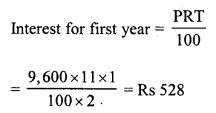Amount after one year = Rs 9,600 + Rs 528 = Rs 10,128
Or principal for second year = Rs 10,128
Interest for the second yearAmount after second year = Rs 10,128 + Rs 557.04 = Rs 10685.04
or Principal for the third year = Rs 10685.04
Interest for the third year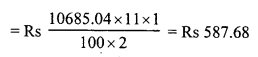Total compound interest = Rs 528 + Rs 557.04 + Rs 587.68 = Rs 1672.72

Question 14.
Surabhi borrowed a sum of Rs 12,000 from a finance company to purchase a refrigerator. If the rate of interest is 5% per annum compounded annually, calculate the compound interest that Surabhi has to pay to the company after 3 years.
Solution:
Sum of money borrowed (P) = Rs 12,000
Rate (R) = 5% p.a.
Period (T) = 3 years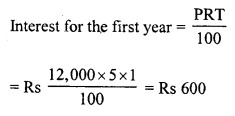Amount after one year = Rs 12,000 + Rs 600 = Rs 12,600
Or principal for the second year = Rs 12,600
Interest for the second year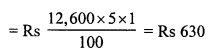Amount after second year = Rs 12,600 + Rs 630 = Rs 13,230
Or Principal for the third year = Rs 13,230
Interest for the third year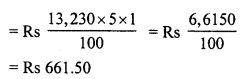Total compound interest for 3 years = Rs 600 + Rs 630 + Rs 661.50 = Rs 1891.50

Question 15.
Daljit received a sum of Rs 40,000 as a loan from a finance company. If the rate of interest is 7% per annum compounded annually, calculate the compound interest that Daljit pays after 2 years.
Solution:
Amount of loan (P) = Rs 40,000
Rate (R) = 7% p.a.
Period = 2 yearsAmount after one year = Rs 40,000 + Rs 2,800 = Rs 42,800
Or principal for the second year = Rs 42,800
Interest for the second year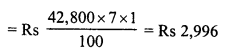Total interest paid after two years = Rs 2,800 + 2,996 = Rs 5,796

Hope given RD Sharma Class 8 Solutions Chapter 14 Compound Interest Ex 14.1 are helpful to complete your math homework.

If you have any doubts, please comment below. Learn Insta try to provide online math tutoring for you.